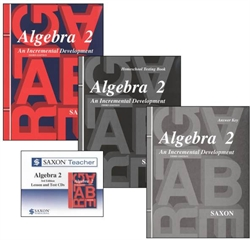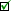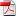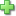# Saxon Algebra 2 - Home School Bundle with Teacher CD

Publisher: Saxon Publishers
3rd Edition, Item: 33111
Curriculum Bundle
List Price: \$208.40 Our Price: \$187.56
Used Price: \$145.88 (2 in stock)Condition Policy

See series description for full review.

Algebra 2 not only treats topics that are traditionally covered in second year algebra, but also a considerable amount of geometry. Specific topics include: graphical solution of simultaneous equations; scientific notation; radicals; roots of quadratic equations; properties of real numbers; inequalities and systems of inequalities; logarithms and antilogarithms; exponential equations; basic trigonometric functions; algebra of polynomials; vectors; polar and rectangular coordinate systems; and a wide spectrum of algebraic word problems. Time is also spent developing geometric concepts and writing proof outlines (the proof coverage is weak). Applications to other subjects such as physics and chemistry, plus "real world" problems, are also covered. (129 lessons)

The Home Study Kit, which contains the student textbook, answer key and test packet, and the Saxon Teacher CD (which contains lecture CDs and the complete solutions) are both included in this bundle, with a 15% discount.

You will need a scientific calculator (Sharp recommended).

## Samples:Table of ContentsLesson 2Lesson 2 page 1Lesson 2 page 2Lesson 35Lesson 106Test 1Answer KeyClick here to write a review